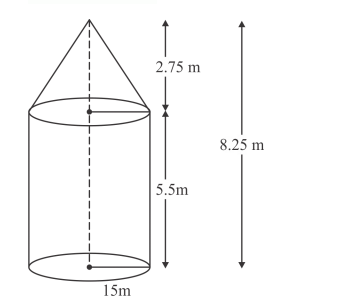# A tent of height 8.25 m is in the form of a right circular cylinderQuestion:

A tent of height 8.25 m is in the form of a right circular cylinder with diameter of base 30 m and height 5.5 m, surmounted by a right circular cone of the same base. Find the cost of the canvas of the tent at the rate of Rs 45 per m2.

Solution:

$l=\sqrt{(2.75)^{2}}+(15)^{2}$

$l=\sqrt{232.5625}$

$=15.25$Total surface area of the tent

$=2 \pi r h+\pi r l$

$=\pi r(2 h+l)$

$=\frac{22}{7} \times 15[2 \times 5-5+15.25]$

$=\frac{330 \times 26.25}{7}$

$=1237.5$

Therefore,

Cost of the canvas of the tent

$=1237.5 \times 45$

$=$ Rs. $55687.50$